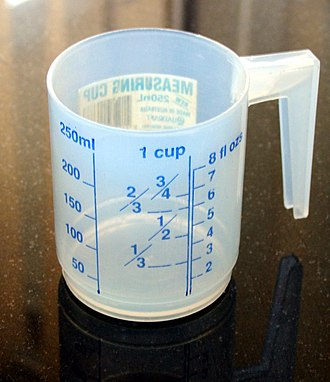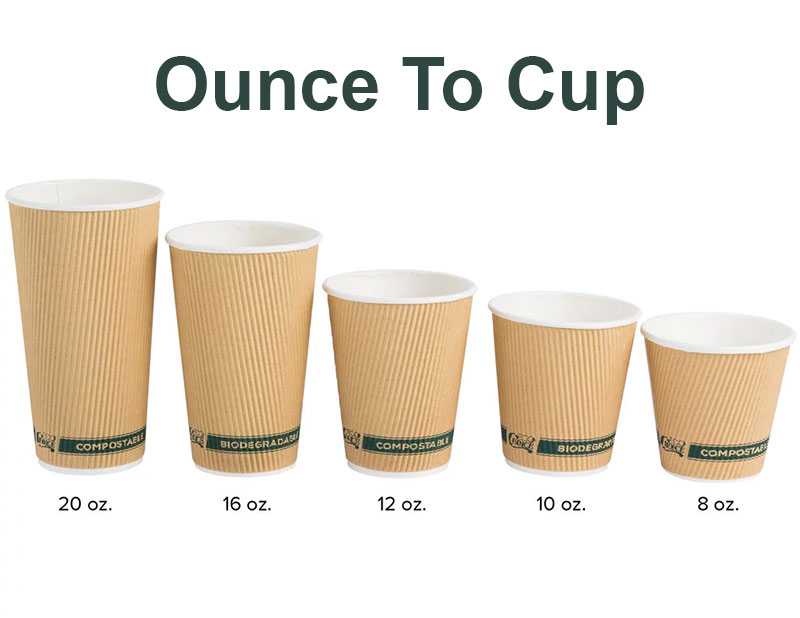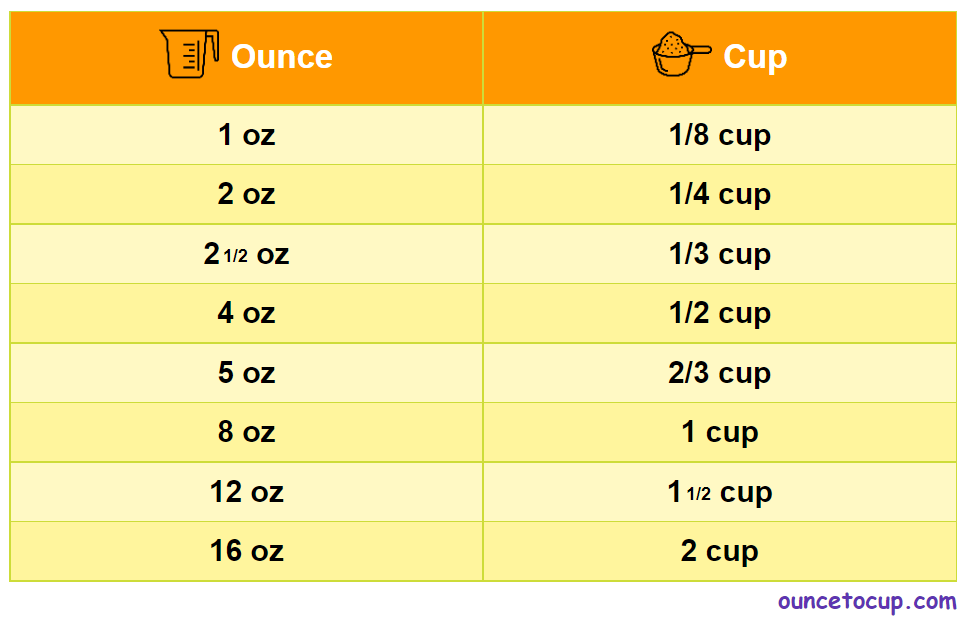# Ounce to Cup Converter (oz to cup)

What makes the dish or your cooking recipe more curious and yummier? Isn’t the actual measurement - YES, with exact measurement on your selected dish-based ingredient brings perfect output. For a delicious dish, an accurate quantity is a major key. If at all you need to know “How many ounces are in a cup” or need a glimpse on how many ounces to cup conversion online chart or any other oz to cups calculations, you have the best source given below and here, we have described detailly about the requirements for your references.

Ounce Value:

Ounces

Cup Value:

Cups

1 Ounce = 0.125 Cup
(1 oz = 0.125 cup)

Try our auto ounce to cup calculator(Without Convert Button), Just change the first field value and you got final value.## How Many Ounces in a Cup?

There is 1 US Cup equal to 8 Ounces. If any of your cooking or baking recipes comes under any cup level measurements and if you don’t have adequate knowledge on using cups – simply, use this Ounce to cup Converter to measure the exact proportion of the leveling compound or mixture.

The Answer is: 8 US Fluid Ounces(8 oz)

1 US Cup = 8 US Fluid Ounce(8 oz to 1 cup)

Note: When you attempt to convert cups and dry ounces, ensure to select an ingredient or specify a density value..

## Oz to Cup:

Ounces to Cup: Ounces can also be mentioned as oz. Do you how many oz makes a Cup?

8 Ounces equals a Cup

Whenever One Cup of liquid or fluid measures, it weighs around 1 Cup which is equal to 8 Ounces. Eventually, you can describe it as 1 Cup = 8 Ounces or 1 Cup = 8 oz (US)## How to convert US Fluid Ounces (oz) to Cups?

It is validated that 1 Fluid Ounce (oz) is capable of measuring 0.125 Cup. It is also alike the calculation of:

• 1 Fluid Ounce (oz) = 0.125 Cup value
• To convert fluid oz to Cups, simply multiply the fluid in ounces i.e. oz by 0.125, or just divide it by 8.
• oz to Cups = number of fluid * 0.125 or
• oz to Cups = numbers of fluids/8

## Some quick table references for fluid ounce to cup conversions:

Ounce [oz] Cup [c]
1 oz0.125 cup
2 oz0.25 cup
3 oz0.375 cup
4 oz0.5 cup
5 oz0.625 cup
6 oz0.75 cup
7 oz0.875 cup
8 oz1 cup
9 oz1.125 cup
10 oz1.25 cup
11 oz1.375 cup
12 oz1.5 cup

## Conversions from Cups to Ounces in another country:

Cups to Ounces Conversion Made in the United States Country

1 US cup = 8 US fluid ounces

1 US cup = 8.327 UK fluid ounces

Cups to ounces conversion made in United Kingdom Recipes

1 UK cup = 10 UK fluid ounces

1 UK cup = 9.607 US fluid ounces

Converting cups to ounces in the rest of the globe

1 global metric cup = 8.454 United States fluid ounces

1 global metric cup = 8.799 United Kingdom fluid ounces

## Now, let’s assume to calculate: how much is 2 ounces in a cup?

• 2 oz to Cups = 2*0.125 = 0.25 Cups
• Or
• 2/8 = 0.25 Cups.
• This is the exact method to calculate or to know/measure oz to Cups.

## How many dry ounces are in a cup?

In general, both dry and liquid measurements are quite similar. Then, 1 dry US cup is equal to 8 fluid ounces (divide the volume value by 8).

## Why Choose us?

Ouncestocup.com delivers an accurate online conversion calculator which includes all type of measurement units. You can get the metric conversion tables for SI units, English units, currency, and other forms of data. If you have conversion types like unit symbols, abbreviations, or full names for units of length, area, mass, pressure, and other types. Examples may include

1. Grams
2. Kilogram
3. Liter
4. Pounds
5. Milliliter
6. Gallon
7. Pint
8. Tablespoon
9. Cubic Centimeter

## Ounces and Cups in Recipes

The ounce (oz) is the direct measurement of mass which is mostly utilized in the UK and the former British colonies, that includes the US, UK, Australia, and New Zealand, among others. It is available in the standardized form in the recent 20th century and is framed in terms of grams (28.35 grams accurately).

The cup is the general measuring unit of volume, with two definitions -which one can be utilized in recipes. The effective usage in US legal cups is they can provide 240 ml of volume. Whereas, the previous units encountered the Imperial cup which equals 284 ml. Hence, you can use our converter space to get your own converted measurement to make your recipes even more delicious.

Preferably, one must not convert weight to volume (oz to cups) in which, the conversion needs some introduction of density via calculation and which can be only done through rough estimates in average. Thus, making use of volumes may feel uncertain in the preparation of a recipe and it is recommended for such measurement activities in the kitchen. Always use kitchen scales to get the best in your food recipes, which can give added essence to sweets and desserts.

## Sixteen Ounces of Milk in Cups

How to convert sixteen ounces of flour to cups, in accordance with the density of flour (0.67) grams per milliliter meaning which shows that the cup holds 5.7 oz of flour.

Solution:

General Formula: oz / 5.7 = cups How the Calculation works: 16 oz / 5.70 = 2.807018 cups Final output: 16 oz is equal to 2.807018 cups

The actual funny part is, we would round up these 2.807018 cups to Three cups of flour while skimming just a bit off the top of the last one.

To be known: You may get rounding errors, so - try to ensure the final results. Utilize this page to study how to convert between ounces and cups easily for your instant recipes.

## Cooking Measurement Conversion TableHence, you may have n-number questions that will strike your mind during your delicious preparations- such repeated questions are answered below:

### FAQ:

#### How big is 48 oz?

A measurement of 48 ounces is equal to 1.36078 Kilograms, 1.41953 liters, or 6 cups.

#### How many cups are in 8 ounces?

1 cup equals 8 ounces.

#### How Many Cups is 13.5 oz?

13.5 ounces is equivalent to 1.69 cups.

#### What is 1/4 a cup in oz?

Here, the measurement of 1/4 cup is equal to 2 fluid ounces.

#### What is 1/8 a cup in oz?

Here, the measurement of 1/8 cup is equal to 1 fluid ounces.

#### What is 1/2 a cup in oz?

Here, the measurement of 1/2 cup is equal to 4 fluid ounces.

#### What is 2/3 a cup in oz?

Here, the measurement of 2/3 cup is equal to 5 fluid ounces.

#### How many cups are 5 ounces of water?

Instant conversion chart of ounces to cups 1 ounce to cups = 0.125 cups, 5 ounces to cups = 0.625 cups, 10 ounces to cups = 1.25 cups. So, try to use the link to get the accurate other related measurements.

#### How many ounces in 1 cubic meter?

Here, the measurement of 1 cubic meter is equal to 35195.079727854 ounces, or 4226.7528198649 cups.

Related ounce conversion calculators: